# RD Sharma Class 8 Solutions Chapter 2 Powers MCQS

In this chapter, we provide RD Sharma Class 8 Solutions Chapter 2 Powers MCQS for English medium students, Which will very helpful for every student in their exams. Students can download the latest RD Sharma Class 8 Solutions Chapter 2 Powers MCQS pdf, free RD Sharma Class 8 Solutions Chapter 2 Powers MCQS Maths book pdf download. Now you will get step by step solution to each question.

### RD Sharma Solutions for Class 8 Chapter 2 Powers MCQS Download PDF

Question 1.
Square of (−23)Solution:Question 2.
Cube of −12 isSolution:Question 3.
Which of the following is not equal toSolution:Question 4.
Which of the following in not reciprocal of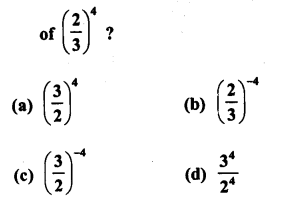Solution: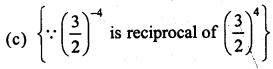Question 5.
Which of the following numbers is not equal to −827 ?Solution:Question 6.Solution:Question 7.Solution:Question 8.Solution:Question 9.Solution:Question 10.Solution:Question 11.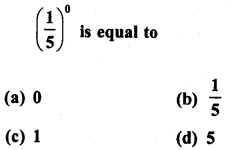Solution: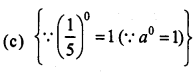Question 12.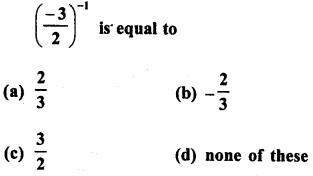Solution:Question 13.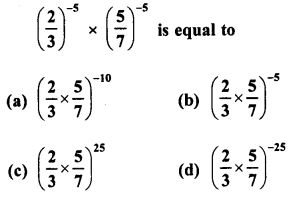Solution:Question 14.Solution:Question 15.
For any two non-zero rational numbers a and b,a4+b4 is equal to
(a) (a + b)1
(b) (a + b)0
(c) (a + b)4
(d) (a + b)8
Solution:
(c) {∵ a4 + b4 = (a + b)4}

Question 16.
For any two rational numbers a and b, a5 x b5 is equal to
(a) (a x b)0
(b) (a x b)10

(c) (a x b)5
(d) (a x b)25

Solution:
(c) {∵ a5 x b5 = (a x b)5}

Question 17.
For a non-zero rational number a, a7 + a12 is equal to
(a) a5
(b) a-19

(c) a-5
(d) a19

Solution:
(c) {a5 a12 = a7-12 =a-5}

Question 18.
For a non-zero rational number a, (a3)-2 is equal to
(a) a6
(b) a-6
(c) a-9
(d) a1

Solution:
(b) {(a3)-2 = a3 x (-2)= a6}

All Chapter RD Sharma Solutions For Class 8 Maths

—————————————————————————–

All Subject NCERT Exemplar Problems Solutions For Class 8

All Subject NCERT Solutions For Class 8

*************************************************

I think you got complete solutions for this chapter. If You have any queries regarding this chapter, please comment on the below section our subject teacher will answer you. We tried our best to give complete solutions so you got good marks in your exam.

If these solutions have helped you, you can also share rdsharmasolutions.in to your friends.